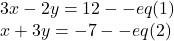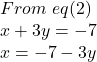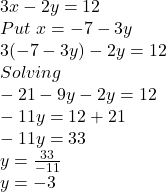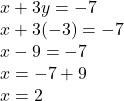## solve this simultaneous equation by using substitution method 3x-2y=12 and x+3y=-7 ​

Question

solve this simultaneous equation by using substitution method 3x-2y=12 and x+3y=-7 ​

in progress 0
1 month 2021-08-15T05:18:50+00:00 1 Answers 1 views 0

Solving this simultaneous equation by using substitution method 3x-2y=12 and x+3y=-7 ​we get x=2 and y=-3

Solution set = {2,-3}

Step-by-step explanation:

We need to solve this simultaneous equation by using substitution method 3x-2y=12 and x+3y=-7 ​

let:Finding value of x from equation 2 and putting in eq(1)Putting value of x in eq(1)So, we get y=-3

Now put value of y in equation 2 to find value of xSo, we get value of x =2

So, solving this simultaneous equation by using substitution method 3x-2y=12 and x+3y=-7 ​we get x=2 and y=-3

Solution set = {2,-3}